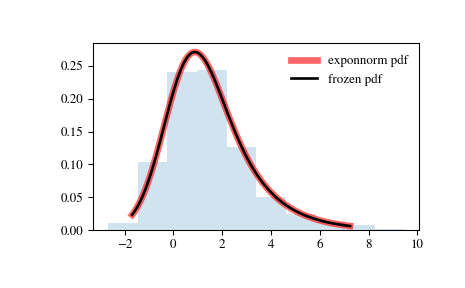# scipy.stats.exponnorm¶

scipy.stats.exponnorm = <scipy.stats._continuous_distns.exponnorm_gen object>[source]

An exponentially modified Normal continuous random variable.

As an instance of the rv_continuous class, exponnorm object inherits from it a collection of generic methods (see below for the full list), and completes them with details specific for this particular distribution.

Notes

The probability density function for exponnorm is:

$f(x, K) = \frac{1}{2K} \exp\left(\frac{1}{2 K^2}\right) \exp(-x / K) \text{erfc}\left(-\frac{x - 1/K}{\sqrt{2}}\right)$

where the shape parameter $$K > 0$$.

It can be thought of as the sum of a normally distributed random value with mean loc and sigma scale and an exponentially distributed random number with a pdf proportional to exp(-lambda * x) where lambda = (K * scale)**(-1).

The probability density above is defined in the “standardized” form. To shift and/or scale the distribution use the loc and scale parameters. Specifically, exponnorm.pdf(x, K, loc, scale) is identically equivalent to exponnorm.pdf(y, K) / scale with y = (x - loc) / scale.

An alternative parameterization of this distribution (for example, in Wikipedia) involves three parameters, $$\mu$$, $$\lambda$$ and $$\sigma$$. In the present parameterization this corresponds to having loc and scale equal to $$\mu$$ and $$\sigma$$, respectively, and shape parameter $$K = 1/(\sigma\lambda)$$.

New in version 0.16.0.

Examples

>>> from scipy.stats import exponnorm
>>> import matplotlib.pyplot as plt
>>> fig, ax = plt.subplots(1, 1)


Calculate a few first moments:

>>> K = 1.5
>>> mean, var, skew, kurt = exponnorm.stats(K, moments='mvsk')


Display the probability density function (pdf):

>>> x = np.linspace(exponnorm.ppf(0.01, K),
...                 exponnorm.ppf(0.99, K), 100)
>>> ax.plot(x, exponnorm.pdf(x, K),
...        'r-', lw=5, alpha=0.6, label='exponnorm pdf')


Alternatively, the distribution object can be called (as a function) to fix the shape, location and scale parameters. This returns a “frozen” RV object holding the given parameters fixed.

Freeze the distribution and display the frozen pdf:

>>> rv = exponnorm(K)
>>> ax.plot(x, rv.pdf(x), 'k-', lw=2, label='frozen pdf')


Check accuracy of cdf and ppf:

>>> vals = exponnorm.ppf([0.001, 0.5, 0.999], K)
>>> np.allclose([0.001, 0.5, 0.999], exponnorm.cdf(vals, K))
True


Generate random numbers:

>>> r = exponnorm.rvs(K, size=1000)


And compare the histogram:

>>> ax.hist(r, density=True, histtype='stepfilled', alpha=0.2)
>>> ax.legend(loc='best', frameon=False)
>>> plt.show()Methods

 rvs(K, loc=0, scale=1, size=1, random_state=None) Random variates. pdf(x, K, loc=0, scale=1) Probability density function. logpdf(x, K, loc=0, scale=1) Log of the probability density function. cdf(x, K, loc=0, scale=1) Cumulative distribution function. logcdf(x, K, loc=0, scale=1) Log of the cumulative distribution function. sf(x, K, loc=0, scale=1) Survival function (also defined as 1 - cdf, but sf is sometimes more accurate). logsf(x, K, loc=0, scale=1) Log of the survival function. ppf(q, K, loc=0, scale=1) Percent point function (inverse of cdf — percentiles). isf(q, K, loc=0, scale=1) Inverse survival function (inverse of sf). moment(n, K, loc=0, scale=1) Non-central moment of order n stats(K, loc=0, scale=1, moments=’mv’) Mean(‘m’), variance(‘v’), skew(‘s’), and/or kurtosis(‘k’). entropy(K, loc=0, scale=1) (Differential) entropy of the RV. fit(data, K, loc=0, scale=1) Parameter estimates for generic data. expect(func, args=(K,), loc=0, scale=1, lb=None, ub=None, conditional=False, **kwds) Expected value of a function (of one argument) with respect to the distribution. median(K, loc=0, scale=1) Median of the distribution. mean(K, loc=0, scale=1) Mean of the distribution. var(K, loc=0, scale=1) Variance of the distribution. std(K, loc=0, scale=1) Standard deviation of the distribution. interval(alpha, K, loc=0, scale=1) Endpoints of the range that contains alpha percent of the distribution

#### Previous topic

scipy.stats.expon

#### Next topic

scipy.stats.exponweib